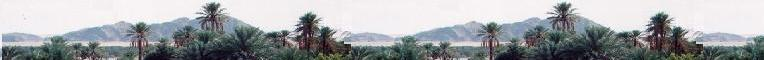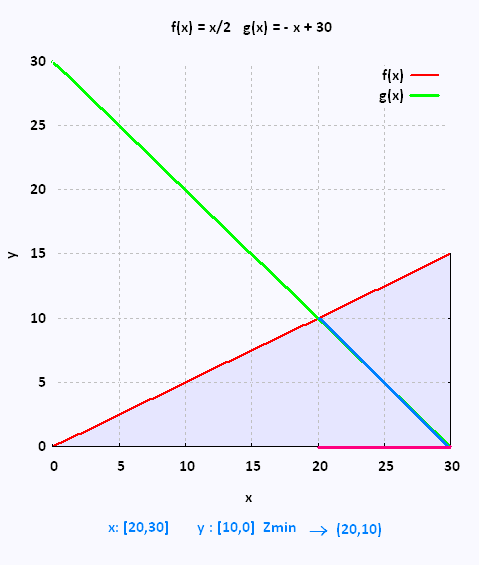# Mathematics 2: Linear optimization Examples

### BooksWe want to buy 30 different books. Books A of Maths at 20$each and and B of Physics at 10$ each. We expect to have at least twice as many books A as books B A ≥ 2B ( greater than twice of B). The money required to buy the books must be the least possible.

If x is the number of Maths books, and
y the number of Physics books,

x ≥ 0
y ≥ 0
x + y = 30
x ≥ 2y
Objective function Z = 20 x + 10 y minimum.

Plot the curves:

D1: y = - x + 30, and
D2: y = x/2The set of solutions is S = [20, 30] for
the x = Maths books, ans S = [10, 0] for the y = Physics Boobs.

Z is minimum

Z = 20 x + 10 y

x = 20, y = 10
Z = 400 + 100 = 500

x = 30, y = 0
Z = 600 + 0 600

x = 25 , y = 5
Z = 500 + 50 = 550

Z min = 500, therefore the unique solution is x = 20 (Maths books), and y = 10 (Physics books).Web ScientificSentence

 chimie labs | scientific sentence | java | php | green cat | contact |